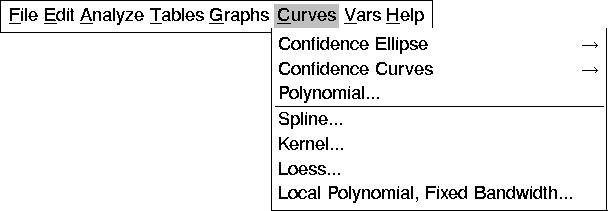Fit Analyses

# Fit Curves

When you are working with one explanatory variable, you can fit curves to the Y-by-X scatter plot generated when the analysis is first created. Use the output dialog (see Figure 39.4, Figure 39.6, and Figure 39.7) or the Curves menu in Figure 39.33 to fit curves to the scatter plot.There are two types of fitting techniques: parametric and nonparametric. Parametric techniques enable you to add confidence ellipses, fit regression polynomials, and add confidence curves of fitted polynomials to the Y-by-X scatter plot. Nonparametric techniques enable you to add spline, kernel, and local polynomial fits to the Y-by-X scatter plot.